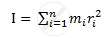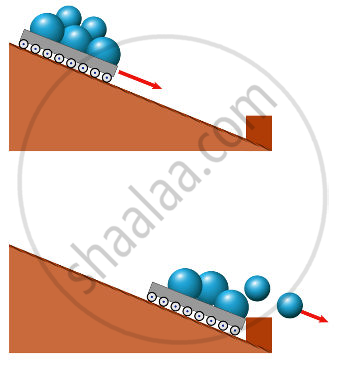Share

# Moment of Inertia

#### notes

Moment of Inertia

• Moment of inertia (I) is analogue of mass in rotational motion.• Moment of inertia about a given axis of rotation resists a change in its rotational motion; it can be regarded as a measure of rotational inertia of the body.

• It is a measure of the way in which different parts of the body are distributed at different distances from the axis.

• the moment of inertia of a rigid body depends on

• The mass of the body,

• Its shape and size

• Distribution of mass about the axis of rotation

• The position and orientation of the axis of rotation.

• The radius of gyration of a body about an axis may be defined as the distance from the axis of a mass point whose mass is equal to the mass of the whole body and whose moment of inertia is equal to the moment of inertia of the body about the axis.

• I = Mk2, where k is radius of gyration.•

### Shaalaa.com

moment of inertia [00:23:03]
S
0%

S